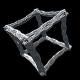# 3D Strange Attractors

After some experimenting, I came up with the following 5 new formulas for generating 3D strange attractors. They all use SIN and COS like Cliff Pickover did when he was creating strange attractors.

All of these formulas were found by trial and error of changing and trying new formulas. In the future it would be interesting to use a genetic/evolutionary system of randomizing formulas and trying to find interesting results automatically.

The x,y and z variables are intialized to 0. Newx, newy and newz are temporary variables to hold the new x,y and z values until the new point is calculated. The parameters in italics a to i are floating point values in the range of -1 to +1 that alter/control the resulting image.

These sample images contain up to one billion plotted points to smooth them out. Each pixel is an average of a super sampled 3×3 grid to help remove aliasing (this is similar to rendering the image at 9 times the size and then downsizing it in an image processing application). The first two samples of each formula are rendered using a z-buffer technique to give them a solid appearance. The third sample of each formula is rendered using an accumulation buffer that shades the pixels based on the number of times each pixel is hit which allows you to see the internal structure of the attractor.

Rampe1 Attractor
newx=z*sin(a*x)+cos(b*y)
newy=x*sin(c*y)+cos(d*z)
newz=y*sin(e*z)+cos(f*x)Rampe2 Attractor
newx=z*sin(a*x)+arccos(b*y)
newy=x*sin(c*y)+arccos(d*z)
newz=y*sin(e*z)+arccos(f*x)Rampe3 Attractor
newx=x*z*sin(a*x)-cos(b*y)
newy=y*x*sin(c*y)-cos(d*z)
newz=z*y*sin(e*z)-cos(f*x)Rampe4 Attractor
newx=x*sin(a*x)+cos(b*y)
newy=y*sin(c*y)+cos(d*z)
newz=z*sin(e*z)+cos(f*x)Rampe5 Attractor
newx=y*sin(a*x)+cos(b*y)+sin(c*z)
newy=z*sin(d*x)+cos(e*y)+sin(f*z)
newz=x*sin(g*x)+cos(h*y)+sin(i*z)All of the above images were created using Visions Of Chaos.

Jason.

## 3 responses to “3D Strange Attractors”

1.Avra says:

Hi! Beautiful shapes!
Did you use Python to create them? Could you give me any script to see how it works? I am a student of Architecture, I just started learning Python and I am interested in creating 3D images of strange attractors..
Also, could you please tell me what are the range of the parameters a, b, c, d, e and f?
Thank you!!

•softologyblog says:

Hi. No I did not use Python.
The parameters a-f are all real values in the range between -1 and +1.

Regards,
Jason.

2.ma5asa says:

Reblogged this on ma5asa and commented:
Rendering Equation and 3D strange attractors